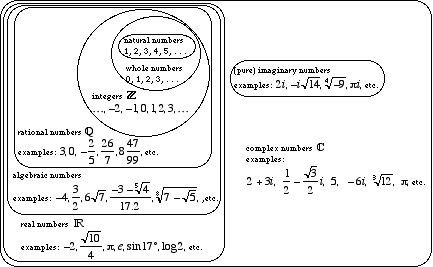# algebraic numbers

Algebraic Numbers

Real numbers that can occur as roots of polynomialequations that have integercoefficients. For example, all rational numbers are algebraic. So are all surds such as $$\sqrt 7$$, as well as numbers built from surds such as the number below.

$\frac{{42 + \sqrt{{15.2}}}}{{\sqrt {4 - \sqrt 3 } }}$.

Note: Real numbers which are not algebraic are known as transcendental numbers.